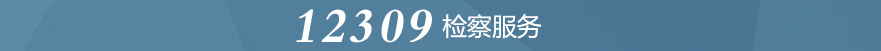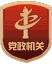﻿

• []
• []
• []
• []
• []
• []
• []

• []
• []
• []
• []
• []
• []
• []

• []
• []

• []
• []
• []
• []
• []
• []
• []• []
• []
• []
• []
• []
• []
• []

• []
• []
• []
• []
• []
• []
• []